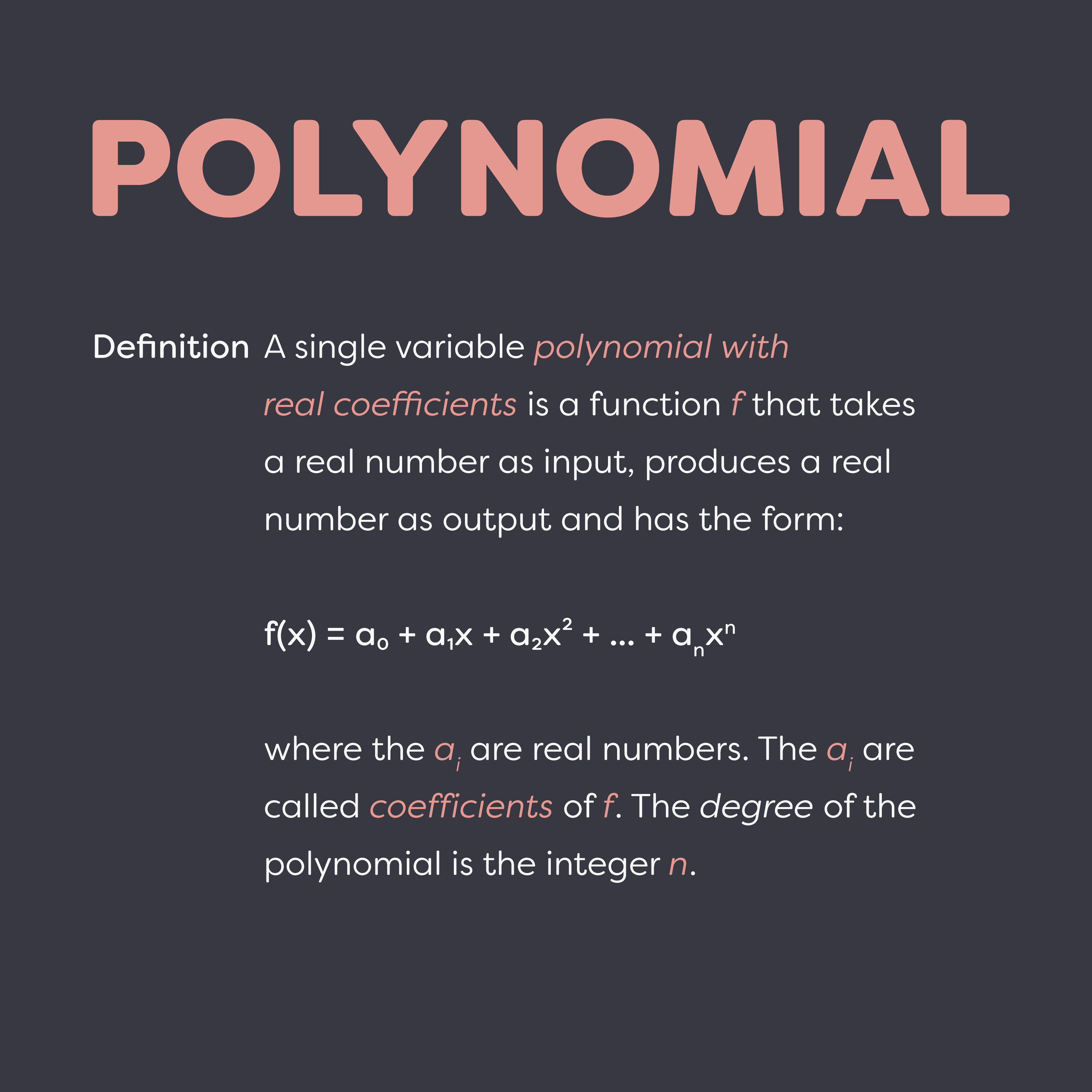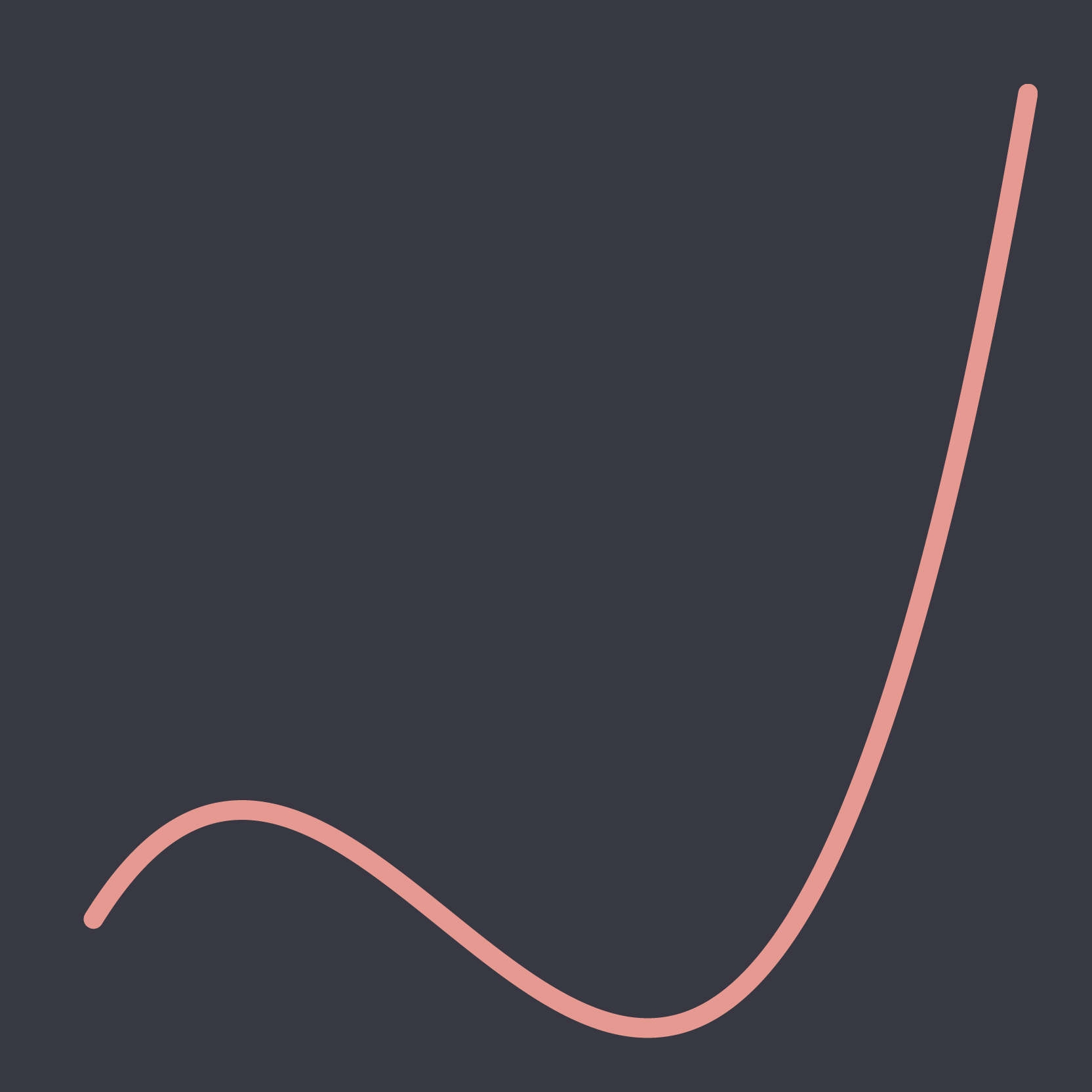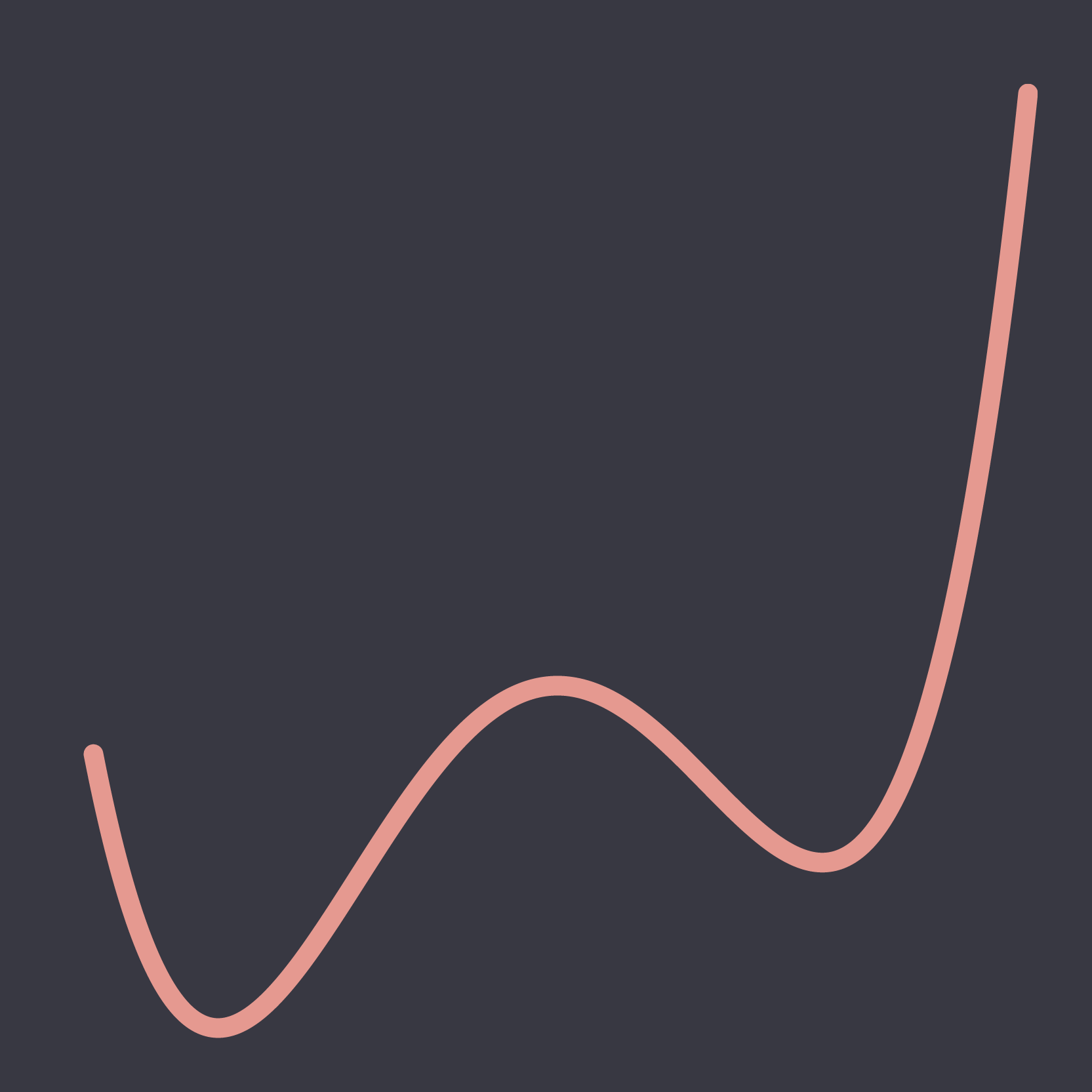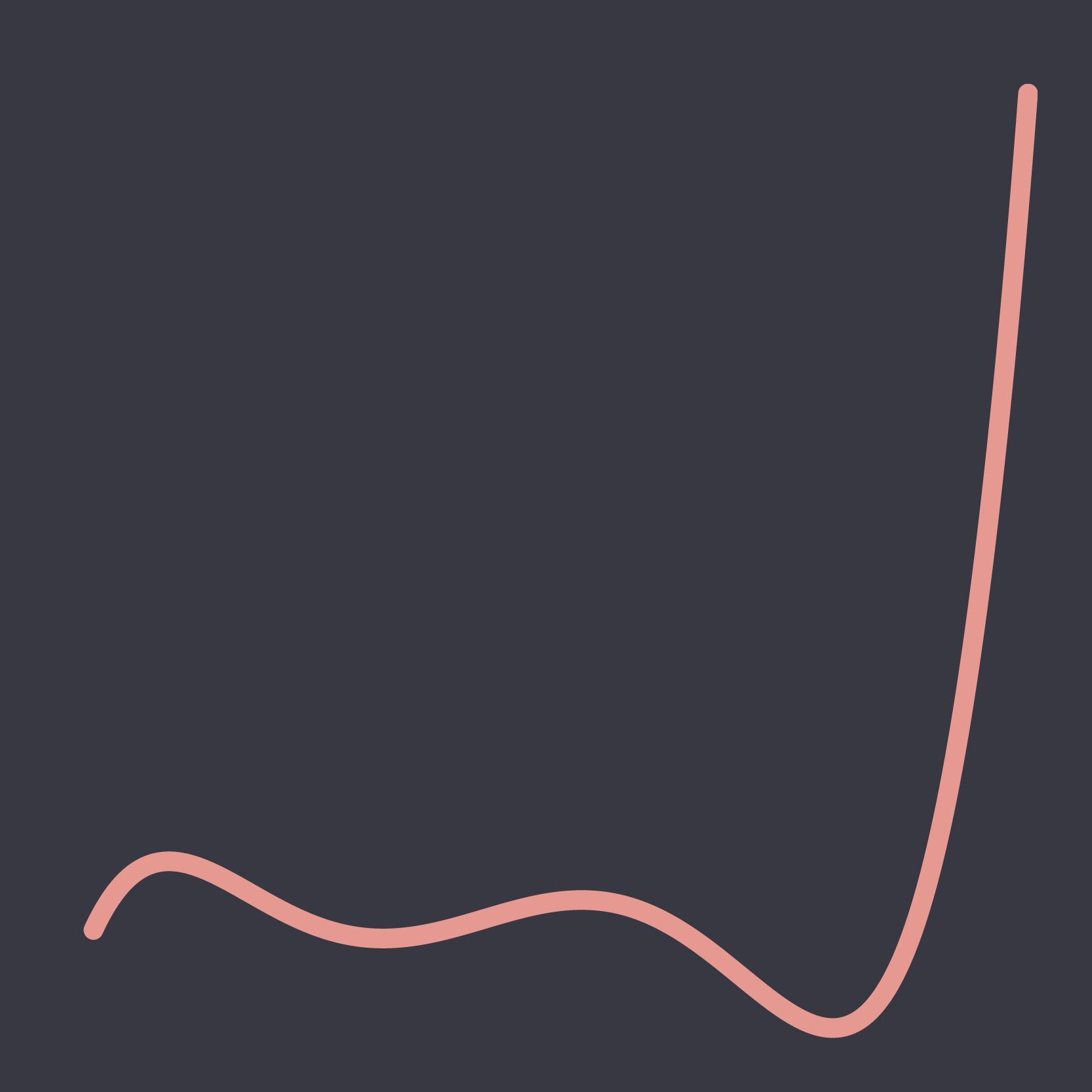# Polynomials

Posted on Jan 07, 2019

For this year one of my goals is to educate myself further in mathematical modeling and machine learning. To kick that off I have started going through the book A Programmer's Introduction to Mathematics. Of course I'm not just gonna read it but I'm also building some visuals to get a better grasp on the concepts.

The book starts with introducing Polynomials so I've made a javascript implementation of a polynomial generator and created some minimalist charts out of it using the React Vis library that I thought to share here.

The main function is this:

``````function polynomial(coefficients) {
return {
degree: coefficients.length,
coefficients: coefficients,
description: function() {
return this.coefficients.reduce((description, coefficient, n) => {
if(coefficient === 0) {
return description
}
let next = description + `\${coefficient}x^\${n}`
if(n < this.coefficients.length-1) {
next += " + "
}
return next
}, 'f(x) = ')
},
calculate: function(x) {
return this.coefficients.reduce((value, coefficient, n) => {
return value + coefficient*Math.pow(x, n)
}, 0)
}
}
}
``````

And I use it like this:

``````// generate a polynomial with coefficients
// -80, -26, 145, 28, -26, -2, 1
let poly = polynomial([-80, -26, 145, 28, -26, -2, 1])
// calculates the function for x = 3.9
console.log(poly.calculate(3.9))
// returns a string with a mathematical description
console.log(poly.description())``````

Try to guess what the coefficients are for each visual ;)Hopefully I'll stay motivated through the year and share more exercices as abstract art later on :)

Code can be found here on my github: https://github.com/dp50mm/Polynomial-Generator

## Subscribe

To stay up to date for new posts.

* indicates required